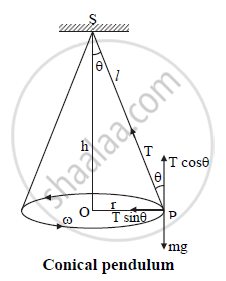HSC Science (Computer Science) 12th Board ExamMaharashtra State Board
Share

Draw a Neat Labelled Diagram of Conical Pendulum. State the Expression for Its Periodic Time in Terms of Length. - HSC Science (Computer Science) 12th Board Exam - Physics

Question

Draw a neat labelled diagram of conical pendulum. State the expression for its periodic time in terms of length.

SolutionS : rigid support

T : tension in the string

l : length of string

h : height of support from bob

v : velocity of bob

r : radius of horizontal circle

θ : semi vertical angle

mg : weight of bob

T=2pisqrt((lcostheta)/g)

This is required expression for time period of conical pendulum in terms of length l.

Is there an error in this question or solution?

APPEARS IN

2014-2015 (October) (with solutions)
Question 3.1 | 2.00 marks

Video TutorialsVIEW ALL 

Solution Draw a Neat Labelled Diagram of Conical Pendulum. State the Expression for Its Periodic Time in Terms of Length. Concept: Periodic and Oscillatory Motions.
S